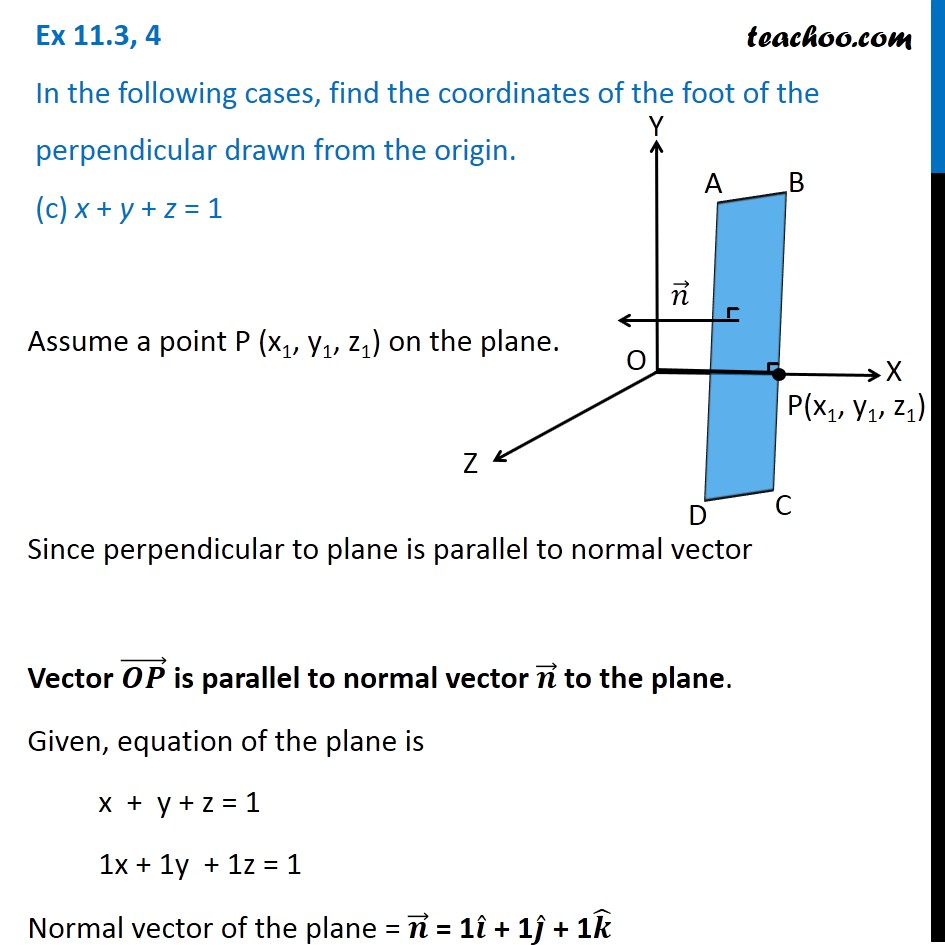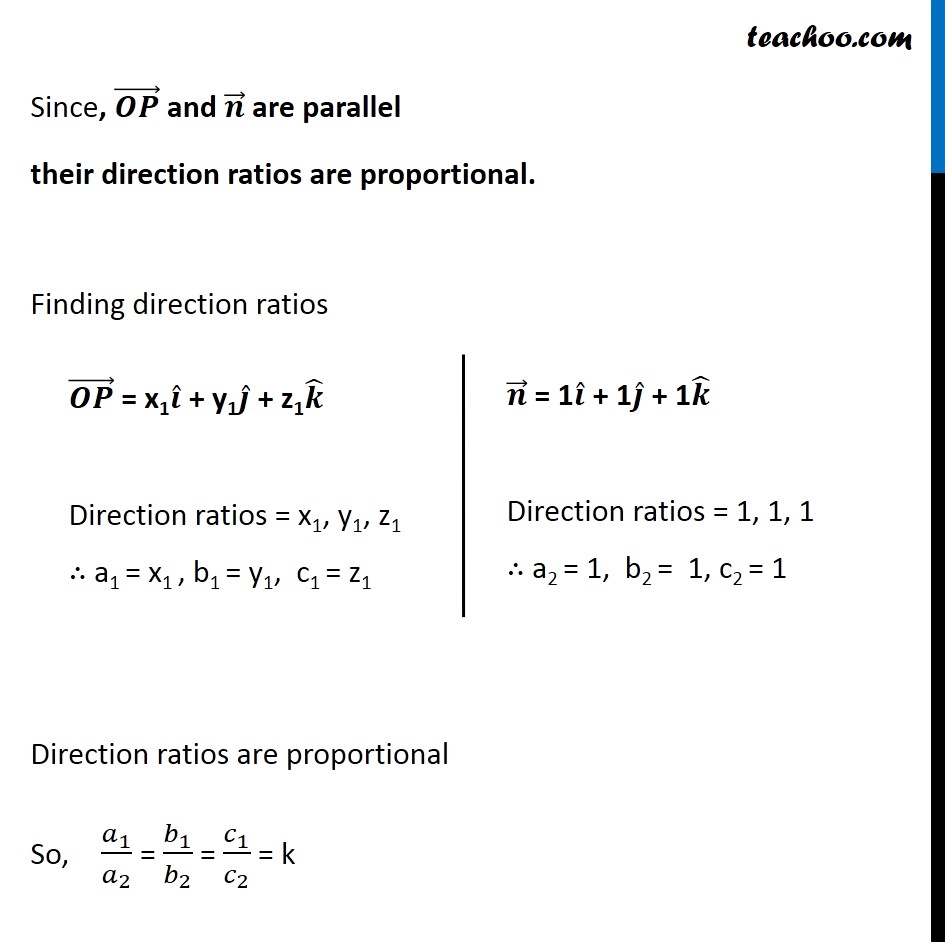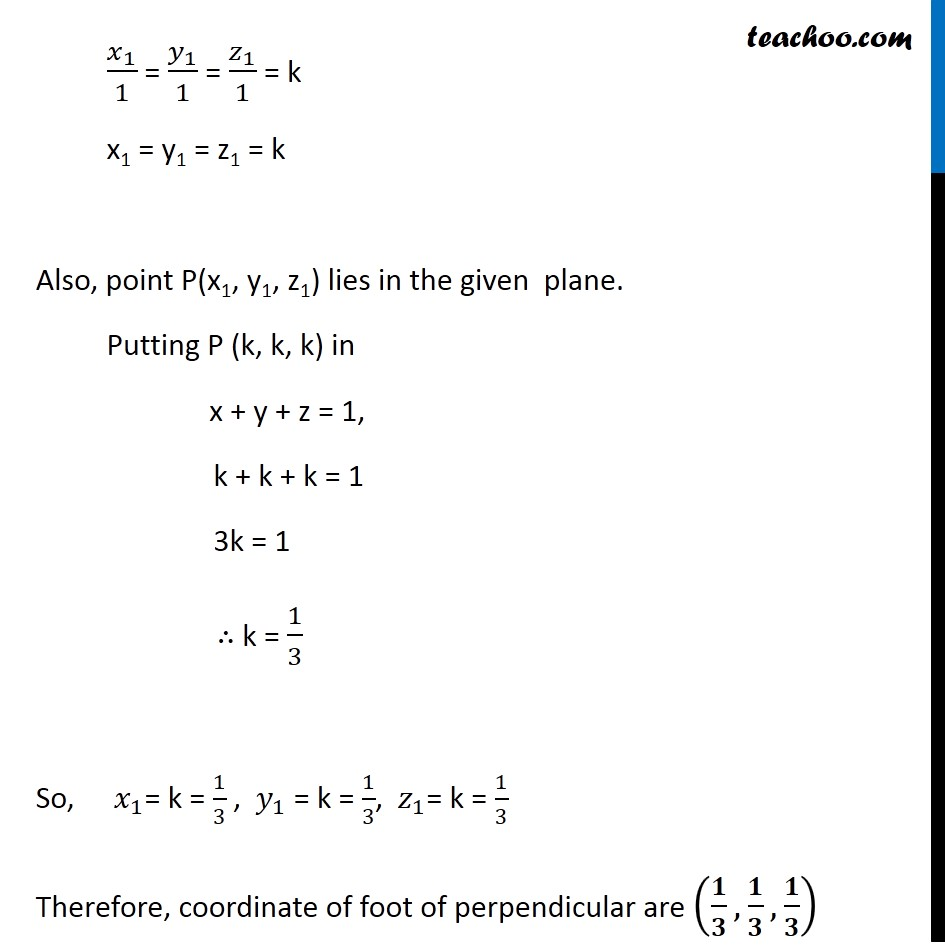Plane

Chapter 11 Class 12 Three Dimensional Geometry
Serial order wiseLearn in your speed, with individual attention - Teachoo Maths 1-on-1 Class

### Transcript

Question 4 In the following cases, find the coordinates of the foot of the perpendicular drawn from the origin. (c) x + y + z = 1 Assume a point P (x1, y1, z1) on the plane. Since perpendicular to plane is parallel to normal vector Vector is parallel to normal vector to the plane. Given, equation of the plane is x + y + z = 1 1x + 1y + 1z = 1 Since, and are parallel their direction ratios are proportional. Finding direction ratios Direction ratios are proportional So, 1 2 = 1 2 = 1 2 = k 1 1 = 1 1 = 1 1 = k x1 = y1 = z1 = k Also, point P(x1, y1, z1) lies in the given plane. Putting P (k, k, k) in x + y + z = 1, k + k + k = 1 3k = 1 k = 1 3 So, 1 = k = 1 3 , 1 = k = 1 3 , 1 = k = 1 3 Therefore, coordinate of foot of perpendicular are , ,